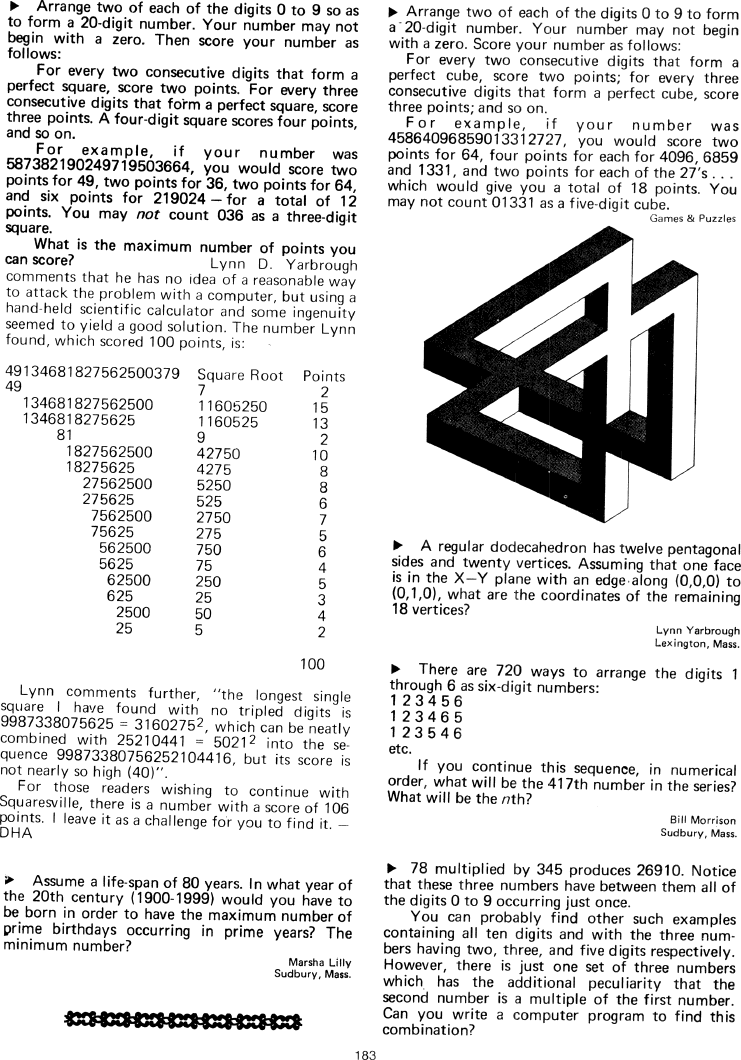The Best of Creative Computing Volume 1 (published 1976)

 `Page 183 << PREVIOUS >> NEXT Jump to page: Go to contents Go to thumbnails`

Puzzles and Problems for Computers and Humans```> Arrange two of each of the digits 0 to 9 so as to form a 20-digit number. Your
number may not begin with a zero. Then score your number as follows:

For every two consecutive digits that form a perfect square, score two points.
For every three consecutive digits that form a perfect square, score three
points. A four-digit square scores four points, and so on.

For example, if your number was 587382190249719503664, you would score two
points for 49, two points for 36, two points for 64, and six points for 219024 -
for a total of 12 points. You may not count 036 as a three-digit square.

What is the maximum number of points you can score? Lynn D. Yarbrough comments
that he has no idea of a reasonable way to attack the problem with a computer,
but using a hand-held scientific calculator and some ingenuity seemed to yield a
good solution. The number Lynn found, which scored 100 points, is:

49134681827562500379 Square Root Points
49 7 2
134681827562500 11605250 15
1346818275625 1160525 13
81 9 2
1827562500 42750 10
18275625 4275 8
27562500 5250 8
275625 525 6
7562500 2750 7
75625 275 5
562500 750 6
5625 75 4
62500 250 5
625 25 3
2500 50 4
25 5 2

100

Lynn comments further, "the longest single square I have found with no tripled
digits is 9987338075625 = 3160275², which can be neatly combined with 25210441
= 5021² into the sequence 99873380756252104416, but its score is not nearly so
high (40)".

For those readers wishing to continue with Squaresville, there is a number with
a score of 106 points. I leave it as a challenge for you to find it. - DHA

> Assume a life-span of 80 years. In what year of the 20th century (1900-1999)
would you have to be born in order to have the maximum number of prime birthdays
occurring in prime years? The minimum number?

Marsha Lilly
Sudbury, Mass.

[image]

> Arrange two of each of the digits 0 to 9 to form a 20-digit number. Your
number may not begin with a zero. Score your number as follows:

For every two consecutive digits that form a perfect cube, score two points; for
every three consecutive digits that form a perfect cube, score three points; and
so on.

For example, if your number was 458640968590133l2727, you would score two points
for 64, four points for each for 4096, 6859 and 1331, and two points for each of
the 27's... which would give you a total of 18 points. You may not count 01331
as a five-digit cube.

Games & Puzzles

[image]

> A regular dodecahedron has twelve pentagonal sides and twenty vertices.
Assuming that one face is in the X-Y plane with an edge along (0,0,0) to
(0,1,0), what are the coordinates of the remaining 18 vertices?

Lynn Yarbrough
Lexington, Mass.

> There are 720 ways to arrange the digits 1 through 6 as six-digit numbers:

1 2 3 4 5 6
1 2 3 4 6 5
1 2 3 5 4 6
etc.

If you continue this sequence, in numerical order, what will be the 417th number
in the series? What will be the nth?

Bill Morrison
Sudbury, Mass.

> 78 multiplied by 345 produces 26910. Notice that these three numbers have
between them all of the digits 0 to 9 occurring just once.

You can probably find other such examples containing all ten digits and with the
three numbers having two, three, and five digits respectively. However, there is
just one set of three numbers which has the additional peculiarity that the
second number is a multiple of the first number. Can you write a computer
program to find this combination?Page 183        << PREVIOUS        >> NEXT        Jump to page:                Go to contents        Go to thumbnails

<!--